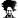# Sum row cells until value is reached

#### absoftver

##### New Member
Hello,

I would really appreciate if someone could help me with example attached.

I need formula that sums up values in row (from column A to column G) while max sum (column I) is less or equal to max value (column H).

Thank you,

Petra

#### Attachments

• 9.2 KB Views: 151

#### Asheesh

##### Excel Ninja
Hi,

Try the below in I2 and drag it down.

MAX((SUBTOTAL(9,OFFSET(\$A\$1,ROW()-1,,,COLUMN(\$A\$2:\$G\$2)))<=\$H2)*SUBTOTAL(9,OFFSET(\$A\$1,ROW()-1,,,COLUMN(\$A\$2:\$G\$2))))

#### r1c1

Staff member
@Asheesh brilliant. I was trying to use SUM(OFFSET(...)) structure and couldn't get it.idea to use SUBTOTAL...

Here is one more:

=LOOKUP(H2, SUBTOTAL(9,OFFSET(A2,,,,COLUMN(\$A\$1:\$G\$1))))

#### Deepak

##### Excel Ninja
Just for fun!

CSE

=SUM(OFFSET(A2,,,,MATCH(H2,SUMIF(OFFSET(A2,,,,COLUMN(\$A\$1:\$G\$1)),">"&0))))

•Asheesh, r1c1 and Khalid NGO

#### Asheesh

##### Excel Ninja
@r1c1 thank you..

That little LOOKUP definitely is icing on the cake..I knew, it could be shortened.. Awesome

#### absoftver

##### New Member
You are amazing! It works great with lookup.

Once again, thank you all very much for your time and help, you saved me lot of nervs. As I am new here, I wonder is it possible to mark this thread as solved for others who might have the same problem?

Petra

#### absoftver

##### New Member
Hm, I just found some mistakes. I'm not sure what goes on here. Any suggestions?

#### Attachments

• 10.2 KB Views: 26

#### Deepak

##### Excel Ninja
Just wrap with IFERROR

#### Asheesh

##### Excel Ninja
Just do as @Deepak suggested..however, you should know that #N/A is not an error or a mistake as per your statement..

Functions like Lookup, vlookup or Match return #N/A if the value you are searching for is not available within the search range..In simple words, it means the value that you are looking for is not present.

#### absoftver

##### New Member
Sorry guys, I just can't get it working.

I am sending my original workbook below. On sheet TEST I need to sum up cells from column E to column AI (for each row from row 5 to row 91) while max sum (column AL) is less or equal to max value (column AJ). In some cases it works, in some not. I really don't know what's the catch.

#### Attachments

• 30.1 KB Views: 48

#### Deepak

##### Excel Ninja
Correct one is ..

=LOOKUP(AJ5, SUBTOTAL(9,OFFSET(E5,,,,COLUMN(\$E\$4:\$AI\$4)-4)))

•Thomas Kuriakose and absoftver

#### Deepak

##### Excel Ninja
Sorry guys, I just can't get it working.

I am sending my original workbook below. On sheet TEST I need to sum up cells from column E to column AI (for each row from row 5 to row 91) while max sum (column AL) is less or equal to max value (column AJ). In some cases it works, in some not. I really don't know what's the catch.

You misunderstood the column part!
It was used to create a array of 1,2....x!

#### absoftver

##### New Member
Yes, it works now without mistakes.Thank you very much for your time and help, I really appreciate it!

I don't use Excel too often, so I am not familiar with arrays, but will definitely take time to study that area for my future work.

Petra

#### DavidC635

##### New Member
@Asheesh brilliant. I was trying to use SUM(OFFSET(...)) structure and couldn't get it.idea to use SUBTOTAL...

Here is one more:

=LOOKUP(H2, SUBTOTAL(9,OFFSET(A2,,,,COLUMN(\$A\$1:\$G\$1))))
Hi

is there a way to count the number of cells/coulmns until that criteria is met instead of a sum?

#### bosco_yip

##### Excel Ninja
Hi

is there a way to count the number of cells/coulmns until that criteria is met instead of a sum?

Try,

=MATCH(H2,INDEX(SUBTOTAL(9,OFFSET(A2,,,,COLUMN(\$A\$1:\$G\$1))),0))

Regards
Bosco

•Thomas Kuriakose

#### DavidC635

##### New Member
Thank you Bosco, works perfectly.
Is it possible to explain why it works? I like to learn about the combination that is used.

thank you again

#### bosco_yip

##### Excel Ninja
Please see that 2 formulas evaluation :

=LOOKUP(H2,SUBTOTAL(9,OFFSET(A2,,,,COLUMN(\$A\$1:\$G\$1))))
>> =LOOKUP(18,{2,10,11,20,20,25,26})
>> =11

=MATCH(H2,INDEX(SUBTOTAL(9,OFFSET(A2,,,,COLUMN(\$A\$1:\$G\$1))),0))
>> =MATCH(18,{2,10,11,20,20,25,26})
>> =3

1] The main core formula is SUBTOTAL+OFFSET() which generate an accumulative sum of A2:G2
=SUBTOTAL(9,OFFSET(A2,,,,COLUMN(\$A\$1:\$G\$1)))
>> ={A2,A2+B2,A2+B2+C2,A2+B2+C2+D2,…......}
>> ={2,2+8,2+8+1,2+8+1+9…......}
>> ={2,10,11,20,20,25,26}

2] The Lookup function based on the criteria, return the closest value (11)

3] The Match function based on the criteria, return the closest value position (3)

Regards
Bosco

Last edited:
•Thomas Kuriakose

#### DavidC635

##### New Member
Thank you Bosco, makes perfect sense.
I am so glad that I found this site

Regards
David

#### Peter Bartholomew

##### Well-Known Member
Letting the headings be built from the number sequence 'k' and the values (row relative) be 'v' the running total 't' can be expressed
= SUMIFS( v, k, "<="&k )
The maximum value subject to the bound 'b' is 'm' which refers to
= MAX( IF( t<=b, t ) )
Similarly, to count the number of terms 'n' that contribute to the total
= COUNT( IF( t<=b, 1 ) )

Since the formulae are all evaluated as named formula, CSE is not needed and the results are
= m and = n,
copied down.

#### Attachments

• 17.7 KB Views: 42
Last edited:
•Thomas Kuriakose

#### Jaff

##### New Member
Please see that 2 formulas evaluation :

=LOOKUP(H2,SUBTOTAL(9,OFFSET(A2,,,,COLUMN(\$A\$1:\$G\$1))))
>> =LOOKUP(18,{2,10,11,20,20,25,26})
>> =11

=MATCH(H2,INDEX(SUBTOTAL(9,OFFSET(A2,,,,COLUMN(\$A\$1:\$G\$1))),0))
>> =MATCH(18,{2,10,11,20,20,25,26})
>> =3

1] The main core formula is SUBTOTAL+OFFSET() which generate an accumulative sum of A2:G2
=SUBTOTAL(9,OFFSET(A2,,,,COLUMN(\$A\$1:\$G\$1)))
>> ={A2,A2+B2,A2+B2+C2,A2+B2+C2+D2,…......}
>> ={2,2+8,2+8+1,2+8+1+9…......}
>> ={2,10,11,20,20,25,26}

2] The Lookup function based on the criteria, return the closest value (11)

3] The Match function based on the criteria, return the closest value position (3)

Regards
Bosco
Is there anyway to get the formula to choose 20? Så the chosen one will be the first number HIGHER than H2?

#### bosco_yip

##### Excel Ninja
Is there anyway to get the formula to choose 20? Så the chosen one will be the first number HIGHER than H2?
It is a 5 years old thread, please open a new post with attachment and example data as well as the expected result

Regards